Question

# Which of the following is soluble in water at 25 degrees * C ? CaSO4 BaSO4...

Which of the following is soluble in water at 25 degrees * C ?
CaSO4
BaSO4
Ag 2SO4
PbSO4
Li2SO4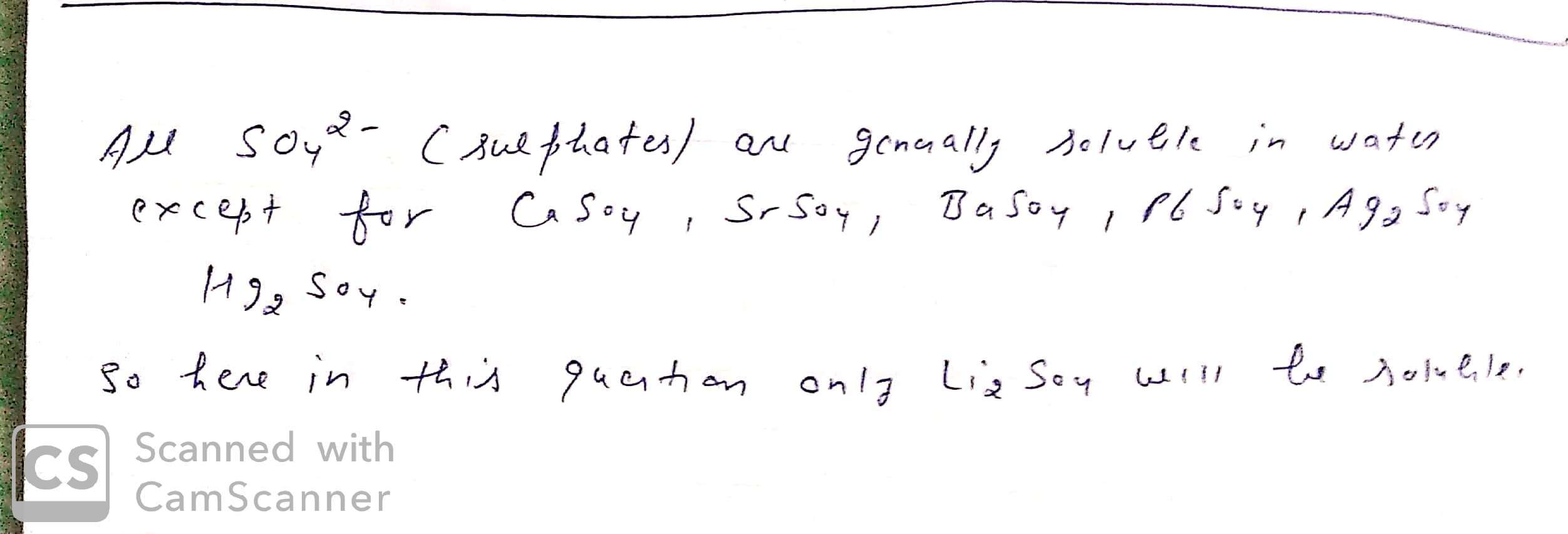#### Earn Coins

Coins can be redeemed for fabulous gifts.

Similar Homework Help Questions
• ### Categorze each of the following ionic compounds as soluble or insoluble in water BaSO4 POE NaNO3...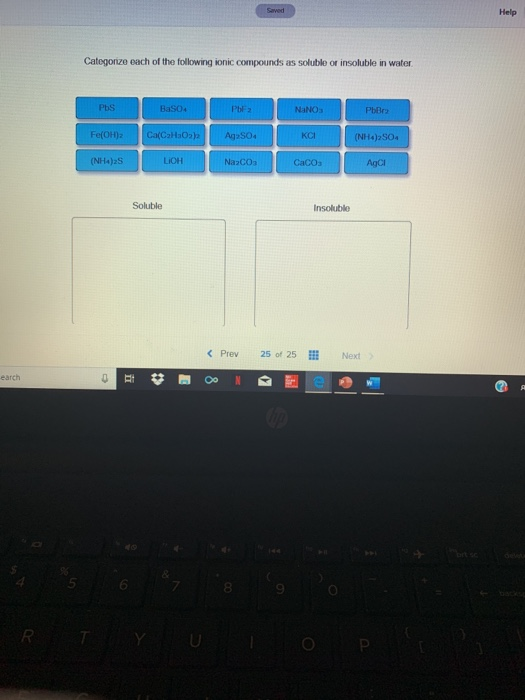Categorze each of the following ionic compounds as soluble or insoluble in water BaSO4 POE NaNO3 PbO Fe(OH)2 CHỌaha0ah Aq2SO4 KC (N) | 42904 (NH) LICH NaCO CaCO3 ACI Soluble Insoluble < Prev 25 of 25 Next

• ### 0.00490 grams of baso4 dissolved in 2.0 liters of water at 25 degrees celcius calculate ksp...

0.00490 grams of baso4 dissolved in 2.0 liters of water at 25 degrees celcius calculate ksp for baso4

• ### Which salts will be more soluble in an acidic solution than in pure water? 0 BaSO4...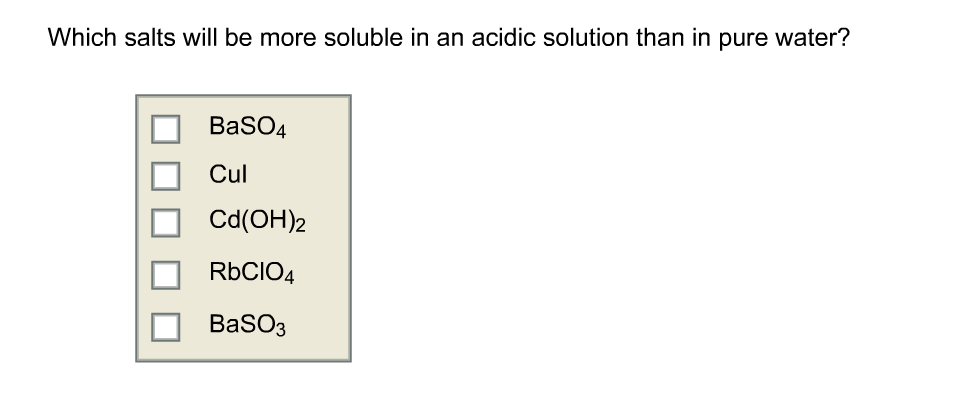Which salts will be more soluble in an acidic solution than in pure water? 0 BaSO4 Cul Cd(OH)2 RbCIO4 BaSO3

• ### how many of the four substances below are considered soluble at 25°C? CuCl2 CaSO4 PbS Ag3PO4...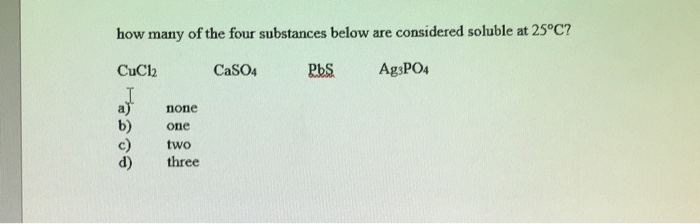how many of the four substances below are considered soluble at 25°C? CuCl2 CaSO4 PbS Ag3PO4 none one two three

• ### 4. Using the table below which give the solubility rules for ionic compounds, predict whether each...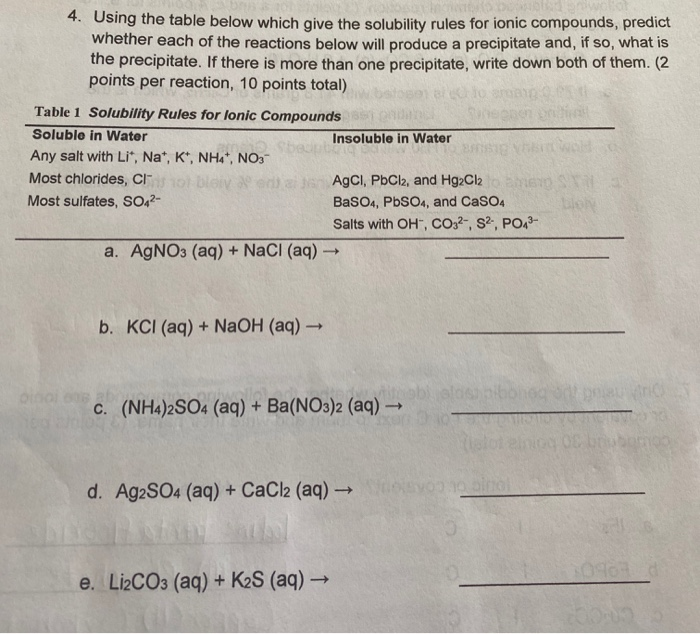4. Using the table below which give the solubility rules for ionic compounds, predict whether each of the reactions below will produce a precipitate and, if so, what is the precipitate. If there is more than one precipitate, write down both of them. (2 points per reaction, 10 points total) Table 1 Solubility Rules for lonic Compounds Soluble in Water Insoluble in Water Any salt with Li+, Na+, K+, NH4+, NO3- Most chlorides, C oblava a AgCl, PbCl2, and HgCl2...

• ### #1,2,3,4,5 state if the following ionic compounds will be soluble or insoluble in water and give...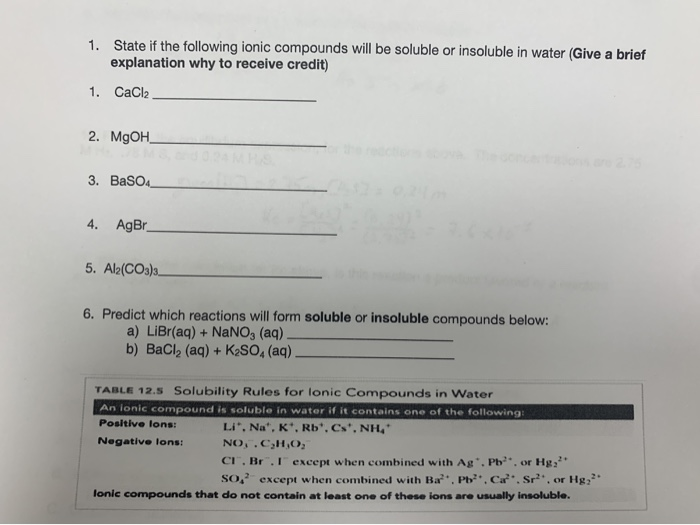#1,2,3,4,5 state if the following ionic compounds will be soluble or insoluble in water and give a brief explanation why. #6 Predict which reactions will form soluble or insoluble compounds: A &B 1. State if the following ionic compounds will be soluble or insoluble in water (Give a brief explanation why to receive credit) 1. CaCl2 2. MgOH 3. BaSO4 4. AgBr 5. Al2(CO3)3. 6. Predict which reactions will form soluble or insoluble compounds below: a) LiBr(aq) + NaNO, (aq)....

• ### The Ksp of Caso4 is 4.93x 10-5 Calculate the solubility (in g/L) of Caso4(s) in 0.250...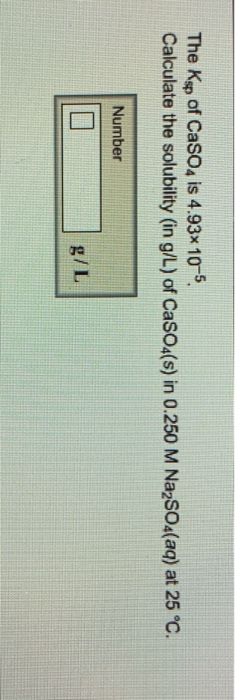The Ksp of Caso4 is 4.93x 10-5 Calculate the solubility (in g/L) of Caso4(s) in 0.250 M NazSO4(aq) at 25 °C. Number g/L Which salts will be more soluble in an acidic solurtion than in pure water? PbCl2 PbSO □ CaCO3

• ### In which of the following pairs of ionic compounds are both chemicals soluble in water? Group...

In which of the following pairs of ionic compounds are both chemicals soluble in water? Group of answer choices LiOH and Mg3(PO4)2 NaCl and KCl PbSO4 and NaCl Na3PO4 and NaCl

• ### Predict whether the compounds are soluble or insoluble in water. AgNO3 CoBr2 AgI K2SO4 NiCO3 BaSO4

Predict whether the compounds are soluble or insoluble in water. AgNO3 CoBr2 AgI K2SO4 NiCO3 BaSO4

• ### QUESTION 10 Which of the following ionic compounds is INSOLUBLE in water? LIHCO3. OK2CO2 Li2SO4 PbSO4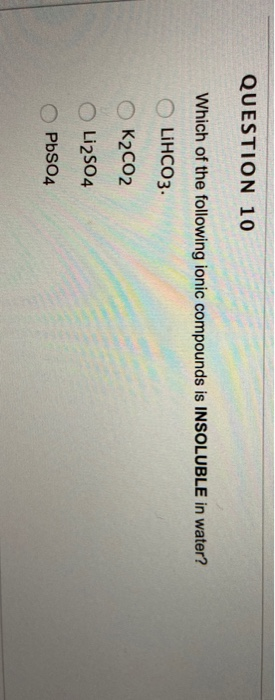QUESTION 10 Which of the following ionic compounds is INSOLUBLE in water? LIHCO3. OK2CO2 Li2SO4 PbSO4